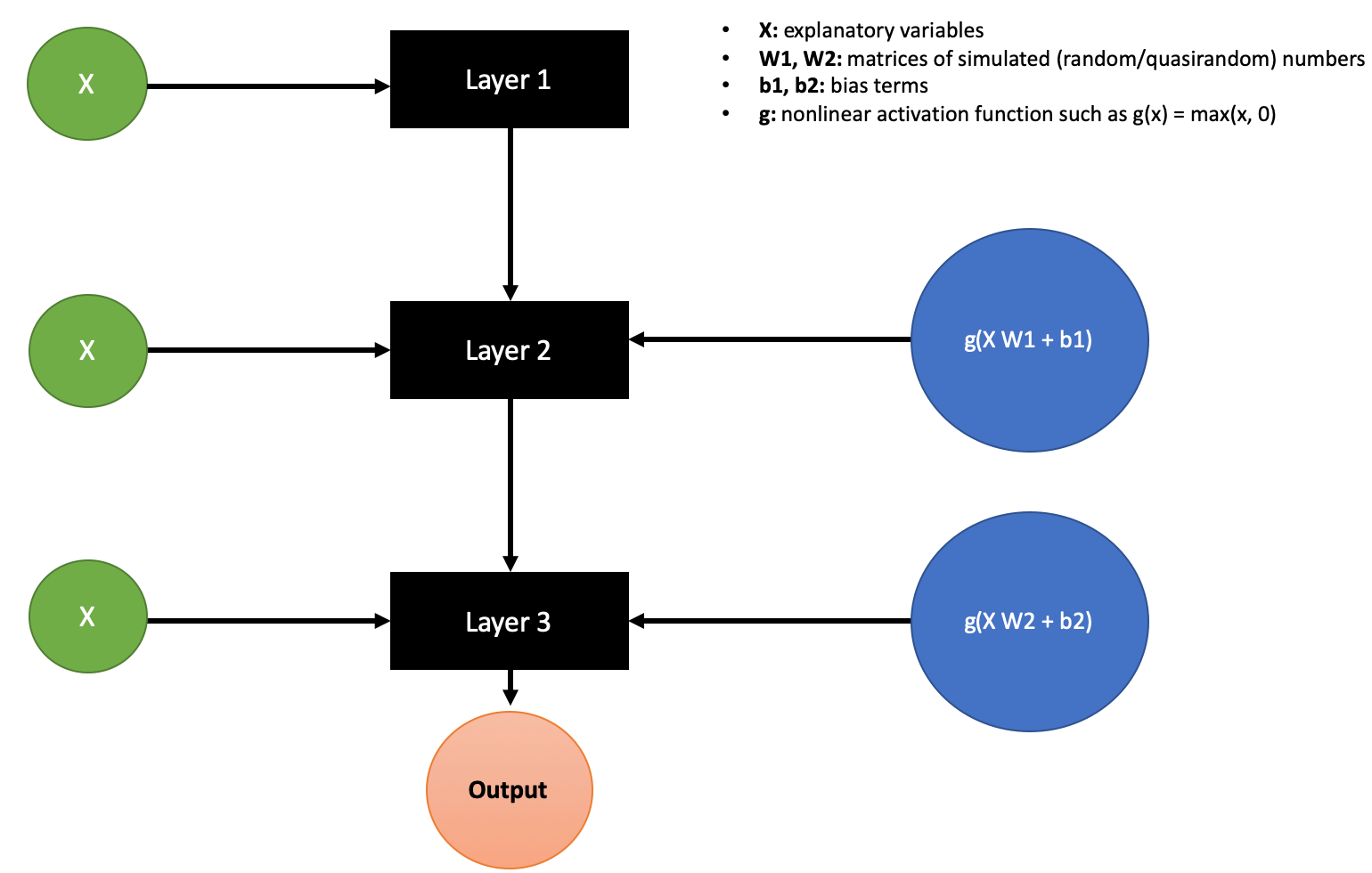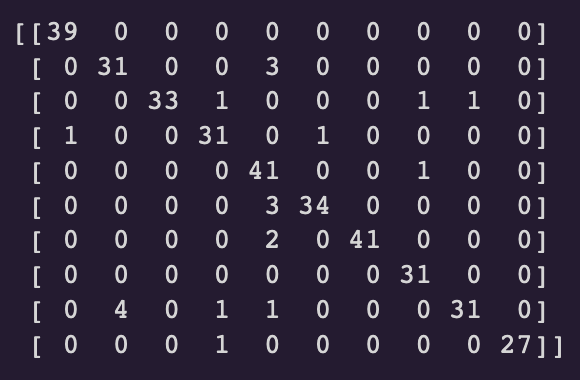As you may know already (or not), nnetsauce contains `CustomClassifier` and `CustomRegressor` models, which allow to derive a new statistical/Machine Learning (ML) model from another one.

Creating this new ML model is achieved by doing some feature engineering. That is, by augmenting the original set of explanatory variables, using random or quasirandom numbers and nonlinear activation functions such as hyperbolic tangent or ReLU.

nnetsauce’s `Custom*`’s can also be chained together, to create a multilayered learning architecture. An example of a 3-layered stack is depicted below, and then demonstrated in an example in Python.# Example in Python

This example is based on a dataset of handwritten digits (0 to 9). The goal is to recognize these digits with nnetsauce,
using a multilayered learning architecture.
We start by installing nnetsauce and importing useful packages for the demo:

## Import packages

```!pip install git+https://github.com/Techtonique/nnetsauce.git
```
```import nnetsauce as ns
from sklearn.ensemble import RandomForestClassifier
from sklearn.model_selection import train_test_split
from time import time
from sklearn.metrics import classification_report
```

## Split data into training and testing sets

```digits = load_digits()
X = digits.data
y = digits.target
X_train, X_test, y_train, y_test = train_test_split(X, y, test_size=0.2,
random_state=123)

```

## Layer 1

Layer 1 is a Random Forest classifier on (X_train, y_train).

```layer1_regr = RandomForestClassifier(n_estimators=10, random_state=123)

start = time()

layer1_regr.fit(X_train, y_train)

# Accuracy in layer 1
print(layer1_regr.score(X_test, y_test))
```## Layer 2 using layer 1

Layer2 is a Random Forest classifier on ([X_train, h_train_1, clusters_1], y_train), where h_train1 = g(X_train W_1 + b_1), and W_1 is simulated (`node_sim`) from a uniform distribution. h_train_1 creates 5 new features (`n_hidden_features`), and 2 indicators of data clusters (`n_clusters`) are added to the set of explanatory variables (clusters_1).

```layer2_regr = ns.CustomClassifier(obj = layer1_regr, n_hidden_features=5,
nodes_sim='uniform', activation_name='relu',
n_clusters=2, seed=123)
layer2_regr.fit(X_train, y_train)

# Accuracy in layer 2
print(layer2_regr.score(X_test, y_test))
```## Layer 3 using layer 2

Layer3 is a Random Forest classifier on ([X_train, h_train_1, clusters_1, h_train_2, clusters_2], y_train) where
h_train2 = g(X_train W_2 + b_2). As in the previous layer, W_2 is simulated from a uniform distribution
h_train2 creates 10 new features and there are 2 additional features (clusters_2), indicators of data clusters. Plus,
70% of nodes in the hidden layer h_train2 are dropped.

```layer3_regr = ns.CustomClassifier(obj = layer2_regr, n_hidden_features=10,
nodes_sim='uniform', activation_name='relu',
n_clusters=2, seed=123)
layer3_regr.fit(X_train, y_train)

# Accuracy in layer 3
print(layer3_regr.score(X_test, y_test))
```The accuracy in layer1, layer2, layer3, are respectively 93.88%, 93.05% and 94.17%. So, in this particular case, if accuracy is the error metric of interest, stacking layers can be useful. Here is a confusion matrix for the whole model, in layer3:

```import matplotlib.pyplot as plt
import seaborn as sns; sns.set()
from sklearn.metrics import confusion_matrix

# model predictions on test set
preds_layer3 = layer3_regr.predict(X_test)

# confusion matrix
mat = confusion_matrix(y_test, preds_layer3)
print(mat)
```